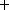Next: An Advanced Example Up: Introduction Previous: Introduction

## General Structure of Input Data

CAIN is not intended for interactive jobs because the computing time is normally more than several minutes. In order to have an idea about the input data structure, let us first give a simple example of an input data. It is a data for an eecollision in a linear collider.

```!  Sample input data for JLC beam-beam interaction.
!  Takes 500 seconds on HP workstation at KEK.
FLAG OFF SPIN;                                       !  Turn off polarization effects
SET   mm=1E-3, micron=1E-6, nm=1E-9,                        !  Define user parameters
ee=250E9,  gamma=ee/Emass,  an=0.65E10,
sigz=0.1*mm, betax=10*mm, betay=100*micron,
emitx=3.3D-6/gamma,  emity=4.8D-8/gamma,
sigx=Sqrt(emitx*betax), sigy=Sqrt(emity*betay),
sigxp=sigx/betax,  sigyp=sigy/betay,  off=sigy*0;
BEAM  RIGHT, KIND=2, NP=10000, AN=an, E0=ee,            !  Define electron beam at IP
BETA=(betax,betay), EMIT=(emitx,emity), SIGT=sigz ;
BEAM  LEFT,  KIND=3, NP=10000, AN=an, E0=ee,            !  Define positron beam at IP
BETA=(betax,betay), EMIT=(emitx,emity), SIGT=sigz ;
SET Smesh=sigz/2;                            !  Longitudinal mesh size for luminosity
! and beam-field calculation.
LUMINOSITY  KIND=(2,3), W=(0,2*1.001*ee,50),   ! Define luminosities to be calculated
WX=8*sigx, WY=8*sigy,  FREP=90*150 ;
LUMINOSITY  KIND=(2,1), W=(0,2*1.001*ee,50),
LUMINOSITY  KIND=(1,1), W=(0,2*1.001*ee,50),
BBFIELD  NX=32, NY=32, WX=8*sigx, R=sigx/sigy/2;             !  Define parameters for
! beam-beam field calculation
CFQED    BEAMSTRAHLUNG;                                      !  Turn on beamstrahlung
FLAG OFF ECHO;
SET it=0;
PUSH  Time=(-2.5*sigz,2.5*sigz,200);                       !  Start tracking
IF it=100;                                !  plot (s,y) profile at 100th time step
PLOT SCAT, KIND=2, H=S/micron, V=Y/nm,
HSCALE=(-250,250), VSCALE=(-12,12),
TITLE='Electron Profile at t=0;',
HTITLE='s(Mm);  G  ;',
VTITLE='y(nm);';
ENDIF;
SET it=it+1;
ENDPUSH;                                                           !  End of tracking
DRIFT S=0;
PRINT STAT;
PLOT  HIST, KIND=1, H=En/1E9, HSCALE=(0,1.001*ee/1E9,50), VLOG,
TITLE='Beamstrahlung Energy Spectrum;',
HTITLE='E0G1  (GeV); XGX       ;';
PLOT  HIST, KIND=(2,3), H=En/1E9, HSCALE=(0,1.001*ee/1E9,50), VLOG,
TITLE='Final Electron Energy Spectrum;',
HTITLE='E0e1  (GeV); X X       ;';
PLOT  SCAT, KIND=2,  MAXNP=2000,
H=Px/Ps/micron, V=Py/Ps/micron,
HSCALE=(-15*sigxp/micron,15*sigxp/micron),
VSCALE=(-5*sigyp/micron,5*sigyp/micron),
TITLE='Final Electron Angle Distribution;',
PLOT LUMINOSITY, VLOG;
WRITE BEAM, KIND=1, FILE=12;
STOP;```

SET command defines various user's variables to be used later.

BEAM commands create right-going electron (KIND=2) and left-going positron (KIND=3) beams with the specified intensity, Twiss parameters, etc.

LUMINOSITY command defines what kind of luminosity (eecenter-of-mass luminosity spectrum in this example) is to be computed.

BBFIELD command defines the parameters (mesh size, etc) for beam-beam field computation, and CFQED command demands the beamstrahlung to be taken into account.

FLAG command controls the echo of the input data.

The pair of PUSH and ENDPUSH commands causes particle tracking step by step for the specified time interval with 200 time steps. At 100th time step, the particle profile is plotted by PRINT SCAT command. This is controled by IF and ENDIF commands.

DRIFT command causes a drift of all the particles onto the reference plane s=0. Then, PRINT STAT prints out the statistical properties (number of particles, r.m.s. size, etc) of all the beams including the beamstrahlung. PLOT HIST commands create a histogram of photon energy in log scale and PLOT LUMINOSITY plots the differential luminosity. WRITE command writes all the photon data on to a file.

STOP command causes stop of CAIN run.

Toshiaki Tauchi
Thu Dec 3 17:27:26 JST 1998